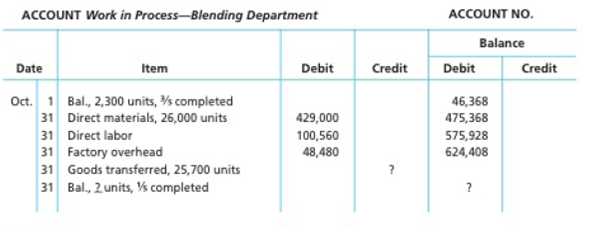# Cost of production report Bavarian Chocolate Company processes chocolate into candy liars. The process begins by placing direct materials (raw chocolate, milk, and sugar) into the Blending Department. All materials are placed into production at the beginning of the blending process. After blending, the milk chocolate is then transferred to the Molding Department, where the milk chocolate is formed into candy bars. The following is a partial work in process account of the Blending Department at October 31: Instructions 1. Prepare a cost of production report and identify the missing amounts for Work in Process—Blending Department. 2. Assuming that the October 1 work in process inventory includes direct materials of $38,295, determine the increase or decrease in the cost per equivalent unit for direct materials and conversion between September and October.BuyFind ### Accounting 27th Edition WARREN + 5 others Publisher: Cengage Learning, ISBN: 9781337272094BuyFind ### Accounting 27th Edition WARREN + 5 others Publisher: Cengage Learning, ISBN: 9781337272094 #### Solutions Chapter Section Chapter 20, Problem 20.2BPR Textbook Problem ## Cost of production reportBavarian Chocolate Company processes chocolate into candy liars. The process begins by placing direct materials (raw chocolate, milk, and sugar) into the Blending Department. All materials are placed into production at the beginning of the blending process. After blending, the milk chocolate is then transferred to the Molding Department, where the milk chocolate is formed into candy bars. The following is a partial work in process account of the Blending Department at October 31:Instructions1. Prepare a cost of production report and identify the missing amounts for Work in Process—Blending Department.2. Assuming that the October 1 work in process inventory includes direct materials of$38,295, determine the increase or decrease in the cost per equivalent unit for direct materials and conversion between September and October.

Expert Solution

(a)

To determine

Process costs

It is a method of cost accounting, which is used where the production is continuous, and the product needs various processes to complete. This method is used to ascertain the cost of the product at each process or stage of production.

Equivalents units for production

The activity of a processing department in terms of fully completed units is known as equivalent units. It includes the completed units of direct materials and conversion cost of beginning work in process, units completed and transferred out, and ending work in process.

Production cost report

A production cost report is a comprehensive report prepared for each department separately at the end of a particular period, which represents the physical flow and cost flow of product for the concerned department.

To Determine: The equivalents units for production of direct materials and conversion costs for the month of October for Company BC.

### Explanation of Solution

Calculate the equivalents units for production of direct materials and conversion costs for the month of October for Company BC as shown below:

Figure (1)

Working notes:

Calculate opening work in process inventory for conversion costs as shown below:

Opening work in process inventory for conversion costs) = 135=25=40%

Calculate units started and completed in October as shown below:

Units started and completed in July = (Units transferred out opening  work in process inventory)=25,700units 2,300units=23,400units

Calculate whole ending work in process inventory in October as shown below:

Whole ending work in process inventory in October)=(Opening work in process inventory +Received from material storeroom Transferred to packing department)=2,300units +26,000units 25,700units=2,600units

Calculate ending work in process inventory for conversion costs as shown below:

Ending work in process inventory for conversion costs) = (Total units for ending work in proces inventory ×Completed percent for conversion costs)=2,600units ×15=520units

Equivalent units for production is calculated by adding units of opening work in process inventory, transferred to finished goods in October, and units for ending work in process inventory. Therefore, an equivalents unit for production for direct materials is 26,000 units and equivalent units for production for conversion costs is 24,840 units.

Calculate direct materials and conversion cost equivalent cost per unit for October as shown below:

Direct material cost per unit =Total direct material costsEquivalent units for direct materials=$429,00026,000units=$16.50Per unit

Conversion cost per unit =Total conversion costsEquivalent units for conversion=$149,04024,840units=$6

Expert Solution

(b)

To determine
Increase or decrease in the cost per equivalent per unit for direct materials and conversion cost between September and October when, work in process inventory as on October 1 includes \$38,295 of direct materials.

### Want to see the full answer?

Check out a sample textbook solution.See solution

### Want to see this answer and more?

Bartleby provides explanations to thousands of textbook problems written by our experts, many with advanced degrees!

See solution